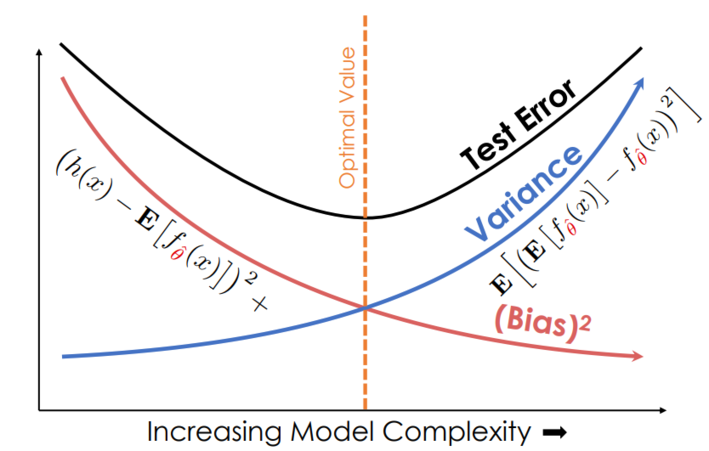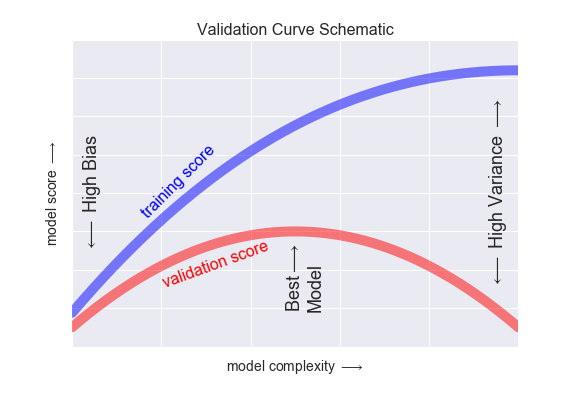# 5.3.1. The objective of machine learning¶

• Prediction accuracy (e.g. of the label or the group detection)

• Feature selection (which X variables and non-linearities should be in the model)

And for both of those, the idea is that what the model learns will work out-of-sample. In the framework of our machine learning workflow, what this means is that after we pick our model in step 5, we only get one chance to apply it on test data and then will move to production models. We want our model to perform as well at step 6 and in production as it does while we train it!

Key takeaway #1

The key to understanding most of the choices you make in a ML project is to remember: The focus of ML is to learn something that generalizes outside of the data we have already!

Econometrically, the goal is to estimate a model on a sample (the data we have) that works on the population (all of the data that can and will be generated in the real world).

A model will create predictions, and those predictions will be wrong to some degree when we generalize outside our initial data.

It turns out we can decompose the expected error of a model like this1

$E[\text{model error risk}] = \text{model bias}^2+\text{model variance}+\text{noise}$

“Model bias”

• Def: Is errors stemming from the model’s assumptions in how it predicts the outcome variable. (It is the opposite of model accuracy.)

• Adding more variables or polynomial transformations of existing variables will usually reduce bias

• Adding more data to the training dataset can (but might not) reduce bias

“Model variance”

• Def: Is extent to which estimated model varies from sample to sample

• Adding more variables or polynomial transformations of existing variables will usually increase model variance

• Adding more data to the training dataset will reduce variance

Noise

• Def: Randomness in the data generating process beyond our understanding

• To reduce the noise term, you need more data, better data collection, and more accurate measurements

Key takeaway #2

We tune the complexity of our models to change our models’ bias and variance, and there is an optimal amount of complexity.

THE FUNDAMENTAL TRADEOFF: Increasing model complexity increases its variance but reduces its bias

• Models that are too simple have high bias but low variance

• Models that are too complex have the opposite problem

• Collecting a TON of data can allow you to use complex models with less variance

This is the essence of the bias-variance tradeoff, a fundamental issue that we face in choosing models for prediction.”2

I like these two graphs to see all of this discussion visually:

• Models that are too simple are said to be “underfit” (take steps to reduce bias)

• Models that are too complicated are said to be “overfit” (take steps to reduce variance)• Models that are too simple perform poorly (low scores, high bias)

• Models that are too complex perform well in training but poorly outside that sample (high variance)Source

Minimizing model risk

Our tools to minimize model risk are

I added some good external resources in the links above on feature engineering and model selection. The next pages here will dig into model evaluation because it gets at the flow of testing a model.

1

(If you want to see the derivation of this, you can go to the wiki page or DS100. The former’s notation is a little simpler but the latter is more helpful with intuition)

2